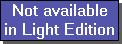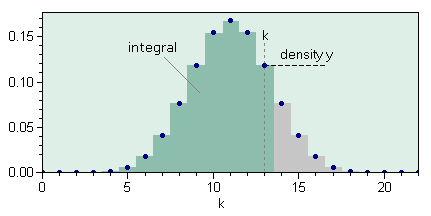The SDL Component Suite is an industry leading collection of components supporting scientific and engineering computing. Please visit the SDL Web site for more information....## BinomDistriDensityUnit: SDL_statis Class: none Declaration: function BinomDistriDensity (SampleSize: integer; FractionA: double; k: integer): double;

The function BinomDistriDensity returns the density value of the binomial distribution for a given quantile k. The parameter SampleSize specifies the size of the drawn sample, the parameter FractionA controls the fraction of items marked "A" (see below). The parameter k may take any value between zero and SampleSize, the parameter FractionA is limited to the interval [0,1]. Both parameters are automatically corrected to the closest limit if an out-of-range value is specified.Background of the binomial distribution: Let's assume to have a container of a huge (infinite) amount of balls with a certain fraction (parameter FractionA) of them being marked by "A", the others being marked by "B". The binomial distribution provides an answer to the question "what is the probability to get k "A" balls when drawing SampleSize balls from the container?".

Last Update: 2020-Aug-04